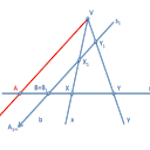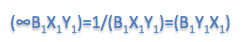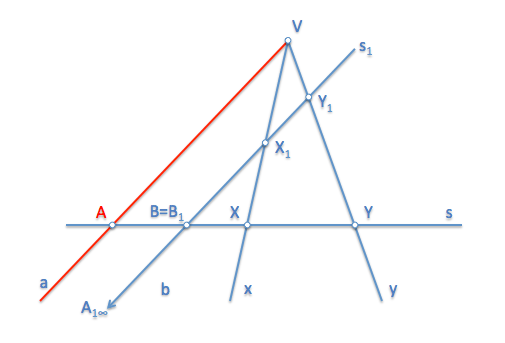# Geometría proyectiva: Construction of quadruples of pointsWe have seen the definition of Elements arranged quaterns, characterizing rectilinear some four points or four straight from a bundle of planes through a value or characteristic, result for the ratio of two triads determined by such elements.

We then consider the problem of obtaining, given three elements belonging to a same form of first category, series or beam, get a fourth element that determines a Tetrad of particular value.

We will first resolve the obtaining of a Tetrad of points, reducing the search of the quadruples of straight lines to the points earned by section of the beams by a straight line.

The wording of the problem can be as follows:

Given three points of a straight line series, set a new point in such a way that the particular Tetrad has a given value. For example (ABXY)= 2/3. In the figure below we see that we must determine the point “A” of the Tetrad (ABXY).Stop solve the problem we must remember that the projection of a point from a vertex V Tetrad determines a Tetrad of straight lines of equal value.If we had A point, We would see that it complies:The vertex V can be any point on the plane which do not belong to the series. Yes this new straight beam is sectioned off by another straight line, s1 for example, We will determine a new Tetrad of points of equal value that formed by the straight lines, and therefore also equal to the one of the points of the original series:This section can be by any line that does not contain a vertex V.

Suppose the particular case in which the straight line which sections to the straight beam is parallel to one of the straight lines, for example the line “to”:In this case, the straight base of the new short series to the straight “to” at infinity. The quadruples of points of the figure shall comply:Since it the terna:It tends to the unit to be the point “A” at infinity.

We see therefore that the Tetrad (ABCD) It can be reduced to a slate of three very special if the section is parallel to the straight line “to” beam. This allows us to minimize the search of a Tetrad of a shortlisting.Once analyzed the problem we can establish a method of resolution for the obtaining of the point “A” of a Tetrad that points are known “B”, “X” and “And”, and the value of the property.About the point “B” We will build a list with the value of the Tetrad that we find, in such a way that we will get part of the elements that we have seen in the previous analysis figures, in particular we will determine the points of the new series section:

The line “s1” on which we have built the three can have any address.

These two series will be prospects that they have a dual element, the “B”, so they will have a Center perspective that relates them:Note that the point “A1” It must be unfit (found in the infinite), so the straight “to” the beam must be parallel to the straight line “s1”. allowing us to determine the point “A” Searches.Can you generalize this construction to find another point of the Tetrad? For example the “B” or “X”